LORENE
Lorene::Eos_tabul Class Referenceabstract

Base class for tabulated equations of state. More...

#include <eos_tabul.h>

Inheritance diagram for Lorene::Eos_tabul: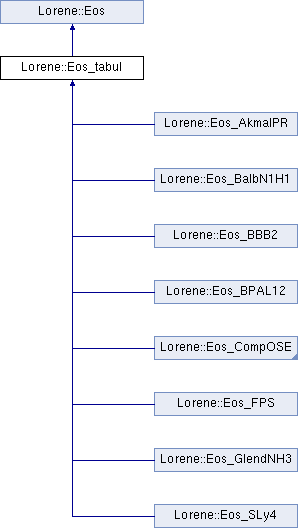## Public Member Functions

virtual ~Eos_tabul ()
Destructor. More...

const string & get_tablename () const

const Tblget_logh () const

double get_hmin () const
Returns minimal value of log-enthalpy in the table. More...

double get_hmax () const
Returns maximal value of log-enthalpy in the table. More...

virtual void sauve (FILE *) const
Save in a file. More...

virtual double nbar_ent_p (double ent, const Param *par=0x0) const
Computes the baryon density from the log-enthalpy. More...

virtual double ener_ent_p (double ent, const Param *par=0x0) const
Computes the total energy density from the log-enthalpy. More...

virtual double press_ent_p (double ent, const Param *par=0x0) const
Computes the pressure from the log-enthalpy. More...

virtual double der_nbar_ent_p (double ent, const Param *par=0x0) const
Computes the logarithmic derivative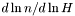from the log-enthalpy. More...

virtual double der_ener_ent_p (double ent, const Param *par=0x0) const
Computes the logarithmic derivative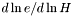from the log-enthalpy. More...

virtual double der_press_ent_p (double ent, const Param *par=0x0) const
Computes the logarithmic derivative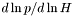from the log-enthalpy. More...

virtual double der_press_nbar_p (double ent, const Param *par=0x0) const
Computes the logarithmic derivative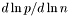from the log-enthalpy. More...

virtual double csound_square_ent_p (double, const Param *) const
Computes the sound speed squared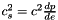from the enthapy with extra parameters (virtual function implemented in the derived classes). More...

const char * get_name () const
Returns the EOS name. More...

void set_name (const char *name_i)
Sets the EOS name. More...

virtual bool operator== (const Eos &) const =0
Comparison operator (egality) More...

virtual bool operator!= (const Eos &) const =0
Comparison operator (difference) More...

virtual int identify () const =0
Returns a number to identify the sub-classe of Eos the object belongs to. More...

Cmp nbar_ent (const Cmp &ent, int nzet, int l_min=0, Param *par=0x0) const
Computes the baryon density field from the log-enthalpy field and extra parameters. More...

Scalar nbar_ent (const Scalar &ent, int nzet, int l_min=0, Param *par=0x0) const
Computes the baryon density field from the log-enthalpy field and extra parameters. More...

Cmp ener_ent (const Cmp &ent, int nzet, int l_min=0, Param *par=0x0) const
Computes the total energy density from the log-enthalpy and extra parameters. More...

Scalar ener_ent (const Scalar &ent, int nzet, int l_min=0, Param *par=0x0) const
Computes the total energy density from the log-enthalpy and extra parameters. More...

Cmp press_ent (const Cmp &ent, int nzet, int l_min=0, Param *par=0x0) const
Computes the pressure from the log-enthalpy and extra parameters. More...

Scalar press_ent (const Scalar &ent, int nzet, int l_min=0, Param *par=0x0) const
Computes the pressure from the log-enthalpy and extra parameters. More...

Cmp der_nbar_ent (const Cmp &ent, int nzet, int l_min=0, Param *par=0x0) const
Computes the logarithmic derivativefrom the log-enthalpy and extra parameters. More...

Scalar der_nbar_ent (const Scalar &ent, int nzet, int l_min=0, Param *par=0x0) const
Computes the logarithmic derivativefrom the log-enthalpy and extra parameters. More...

Cmp der_ener_ent (const Cmp &ent, int nzet, int l_min=0, Param *par=0x0) const
Computes the logarithmic derivativefrom the log-enthalpy and extra parameters. More...

Scalar der_ener_ent (const Scalar &ent, int nzet, int l_min=0, Param *par=0x0) const
Computes the logarithmic derivativefrom the log-enthalpy and extra parameters. More...

Cmp der_press_ent (const Cmp &ent, int nzet, int l_min=0, Param *par=0x0) const
Computes the logarithmic derivativefrom the log-enthalpy and extra parameters. More...

Scalar der_press_ent (const Scalar &ent, int nzet, int l_min=0, Param *par=0x0) const
Computes the logarithmic derivativefrom the log-enthalpy and extra parameters. More...

Scalar csound_square_ent (const Scalar &ent, int nzet, int l_min=0, Param *par=0x0) const
Computes the sound speed squaredfrom the enthalpy with extra parameters. More...

## Static Public Member Functions

static Eoseos_from_file (FILE *)
Construction of an EOS from a binary file. More...

static Eoseos_from_file (ifstream &)
Construction of an EOS from a formatted file. More...

## Protected Member Functions

Eos_tabul (const char *name_i, const char *table, const char *path)
Standard constructor. More...

Eos_tabul (const char *name_i, const char *file_name)
Standard constructor from the full filename. More...

Eos_tabul (const Eos_tabul &)
Copy constructor. More...

Eos_tabul (const char *name_i)
Standard constructor with a name. More...

Eos_tabul (FILE *)
Constructor from a binary file (created by the function sauve(FILE*) ). More...

Eos_tabul (ifstream &ist, const char *table)
Constructor from a formatted file. More...

Eos_tabul (ifstream &ist)
Constructor from a formatted file. More...

Reads the file containing the table and initializes in the arrays logh , logp and dlpsdlh . More...

virtual ostream & operator>> (ostream &) const =0
Operator >> More...

void calcule (const Cmp &thermo, int nzet, int l_min, double(Eos::*fait)(double, const Param *) const, Param *par, Cmp &resu) const
General computational method for Cmp 's. More...

void calcule (const Scalar &thermo, int nzet, int l_min, double(Eos::*fait)(double, const Param *) const, Param *par, Scalar &resu) const
General computational method for Scalar 's. More...

## Protected Attributes

string tablename
Name of the file containing the tabulated data. More...

string authors
Authors - reference for the table. More...

double hmin
Lower boundary of the enthalpy interval. More...

double hmax
Upper boundary of the enthalpy interval. More...

Tbllogh
Table of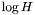. More...

Tbllogp
Table of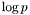. More...

Tbldlpsdlh
Table of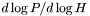. More...

Tbllognb
Table of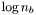. More...

Tbldlpsdlnb
Table of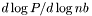. More...

Tbllog_cs2
Table of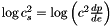. More...

double * press

double * nb

double * ro

char name 
EOS name. More...

## Friends

EosEos::eos_from_file (FILE *)
The construction functions from a file. More...

EosEos::eos_from_file (ifstream &)

## Detailed Description

Base class for tabulated equations of state.

()

EoS data are to be stored in a formatted file in the following format. The first five lines are comments, preceded by hashes. Then is given the number of data lines. After, three other lines of comments, data are given in four columns. The first one is just a number, not used by Lorene. Second is the baryon number density in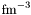, then is the total energy density (including rest mass) and pressure, both in cgs units. Here is an example from the file eos_fps.d:

#  Date: Tue, 21 Nov 2000 17:24:31 +0100
#  From: xxx
#  FPS e.o.s.:   BPS below n.drip, then FPS. Supplied by N.Stergioulas
#  (June 1998) crust bottom at n=0.0957 , rho=1.60E14 1.E50,1.60E14
#
129  <-- Number of lines
#
#            n_B [fm^{-3}]       rho [g/cm^3]        p [dyn/cm^2]
#
6     0.2722041559E-13    0.4518590000E+02    0.1701030000E+15
7     0.1279071657E-12    0.2123260000E+03    0.5817150000E+16  

Note that units used in the table file are not those of Lorene. The interpolation through the tables is a cubic Hermite interpolation, which is thermodynamically consistent, i.e. preserves the Gibbs-Duhem relation. It is defined in [Nozawa, Stergioulas, Gourgoulhon & Eriguchi, Astron. Astrophys. Suppl. Ser. 132 , 431 (1998)], and derives from a general technique presented in [Swesty, J. Comp. Phys. 127 , 118 (1996)].

Definition at line 185 of file eos_tabul.h.

## ◆ Eos_tabul() [1/7]

 Lorene::Eos_tabul::Eos_tabul ( const char * name_i, const char * table, const char * path )
protected

Standard constructor.

Parameters
 name_i Name of the equation of state table Name of the file containing the EOS table path Path to the directory containing the EOS file

Definition at line 164 of file eos_tabul.C.

## ◆ Eos_tabul() [2/7]

 Lorene::Eos_tabul::Eos_tabul ( const char * name_i, const char * file_name )
protected

Standard constructor from the full filename.

Parameters
 name_i Name of the equation of state table Full name of the file containing the EOS table (including the absolute path).

Definition at line 176 of file eos_tabul.C.

## ◆ Eos_tabul() [3/7]

 Lorene::Eos_tabul::Eos_tabul ( const Eos_tabul & )
protected

Copy constructor.

## ◆ Eos_tabul() [4/7]

 Lorene::Eos_tabul::Eos_tabul ( const char * name_i )
protected

Standard constructor with a name.

Definition at line 222 of file eos_tabul.C.

## ◆ Eos_tabul() [5/7]

 Lorene::Eos_tabul::Eos_tabul ( FILE * fich )
protected

Constructor from a binary file (created by the function sauve(FILE*) ).

This constructor is protected because any EOS construction from a binary file must be done via the function Eos::eos_from_file(FILE*) .

Definition at line 188 of file eos_tabul.C.

## ◆ Eos_tabul() [6/7]

 Lorene::Eos_tabul::Eos_tabul ( ifstream & ist, const char * table )
protected

Constructor from a formatted file.

This constructor is protected because any EOS construction from a formatted file must be done via the function Eos::eos_from_file(ifstream& ) .

Parameters
 ist input file stream containing a name as first line and the path to the directory containing the EOS file as second line table Name of the file containing the EOS table

Definition at line 202 of file eos_tabul.C.

## ◆ Eos_tabul() [7/7]

 Lorene::Eos_tabul::Eos_tabul ( ifstream & ist )
protected

Constructor from a formatted file.

This constructor is protected because any EOS construction from a formatted file must be done via the function Eos::eos_from_file(ifstream& ) .

Parameters
 ist input file stream containing a name as first line and the full filename (including the path) containing the EOS file as second line table Name of the file containing the EOS table

Definition at line 213 of file eos_tabul.C.

## ◆ ~Eos_tabul()

 Lorene::Eos_tabul::~Eos_tabul ( )
virtual

Destructor.

Definition at line 232 of file eos_tabul.C.

References dlpsdlh, dlpsdlnb, log_cs2, logh, lognb, and logp.

## ◆ calcule() [1/2]

 void Lorene::Eos::calcule ( const Cmp & thermo, int nzet, int l_min, double(Eos::*)(double, const Param *) const fait, Param * par, Cmp & resu ) const
protectedinherited

General computational method for Cmp 's.

Parameters
 thermo [input] thermodynamical quantity (for instance the enthalpy field)from which the thermodynamical quantity resu is to be computed. nzet [input] number of domains where resu is to be computed. l_min [input] index of the innermost domain is which resu is to be computed [default value: 0]; resu is computed only in domains whose indices are in [l_min,l_min+nzet-1] . In the other domains, it is set to zero. fait [input] pointer on the member function of class Eos which performs the pointwise calculation. par possible extra parameters of the EOS resu [output] result of the computation.

Definition at line 213 of file eos.C.

References Lorene::Cmp::get_etat().

## ◆ calcule() [2/2]

 void Lorene::Eos::calcule ( const Scalar & thermo, int nzet, int l_min, double(Eos::*)(double, const Param *) const fait, Param * par, Scalar & resu ) const
protectedinherited

General computational method for Scalar 's.

Parameters
 thermo [input] thermodynamical quantity (for instance the enthalpy field)from which the thermodynamical quantity resu is to be computed. nzet [input] number of domains where resu is to be computed. l_min [input] index of the innermost domain is which resu is to be computed [default value: 0]; resu is computed only in domains whose indices are in [l_min,l_min+nzet-1] . In the other domains, it is set to zero. fait [input] pointer on the member function of class Eos which performs the pointwise calculation. par possible extra parameters of the EOS resu [output] result of the computation.

Definition at line 285 of file eos.C.

References Lorene::Scalar::get_etat().

## ◆ csound_square_ent()

 Scalar Lorene::Eos::csound_square_ent ( const Scalar & ent, int nzet, int l_min = 0, Param * par = 0x0 ) const
inherited

Computes the sound speed squaredfrom the enthalpy with extra parameters.

Parameters
 ent [input, unit: c^2] enthalpy nzet number of domains where the derivative dln(e)/dln(H) is to be computed. l_min index of the innermost domain is which the coefficient dln(n)/dln(H) is to be computed [default value: 0]; the derivative dln(e)/dln(H) is computed only in domains whose indices are in [l_min,l_min+nzet-1] . In the other domains, it is set to zero. par possible extra parameters of the EOS
Returns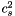[unit: c^2]

Definition at line 499 of file eos.C.

## ◆ csound_square_ent_p()

 double Lorene::Eos_tabul::csound_square_ent_p ( double ent, const Param * ) const
virtual

Computes the sound speed squaredfrom the enthapy with extra parameters (virtual function implemented in the derived classes).

Parameters
 ent [input, unit: c^2] enthalpy par possible extra parameters of the EOS
Returns[unit: c^2]

Implements Lorene::Eos.

Reimplemented in Lorene::Eos_consistent.

Definition at line 599 of file eos_tabul.C.

References Lorene::Tbl::get_taille(), hmax, hmin, Lorene::log10(), log_cs2, logh, lognb, and Lorene::pow().

## ◆ der_ener_ent() [1/2]

 Cmp Lorene::Eos::der_ener_ent ( const Cmp & ent, int nzet, int l_min = 0, Param * par = 0x0 ) const
inherited

Computes the logarithmic derivativefrom the log-enthalpy and extra parameters.

Parameters
 ent [input, unit: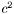] log-enthalpy H defined by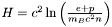, where e is the (total) energy density, p the pressure, n the baryon density, and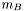the baryon mass nzet number of domains where the derivative dln(e)/dln(H) is to be computed. l_min index of the innermost domain is which the coefficient dln(n)/dln(H) is to be computed [default value: 0]; the derivative dln(e)/dln(H) is computed only in domains whose indices are in [l_min,l_min+nzet-1] . In the other domains, it is set to zero. par possible extra parameters of the EOS
Returns
dln(e)/dln(H)

Definition at line 454 of file eos.C.

## ◆ der_ener_ent() [2/2]

 Scalar Lorene::Eos::der_ener_ent ( const Scalar & ent, int nzet, int l_min = 0, Param * par = 0x0 ) const
inherited

Computes the logarithmic derivativefrom the log-enthalpy and extra parameters.

Parameters
 ent [input, unit:] log-enthalpy H defined by, where e is the (total) energy density, p the pressure, n the baryon density, andthe baryon mass nzet number of domains where the derivative dln(e)/dln(H) is to be computed. l_min index of the innermost domain is which the coefficient dln(n)/dln(H) is to be computed [default value: 0]; the derivative dln(e)/dln(H) is computed only in domains whose indices are in [l_min,l_min+nzet-1] . In the other domains, it is set to zero. par possible extra parameters of the EOS
Returns
dln(e)/dln(H)

Definition at line 464 of file eos.C.

## ◆ der_ener_ent_p()

 double Lorene::Eos_tabul::der_ener_ent_p ( double ent, const Param * par = 0x0 ) const
virtual

Computes the logarithmic derivativefrom the log-enthalpy.

Parameters
 ent [input, unit:] log-enthalpy H
Returns
dln(e)/dln(H)

Implements Lorene::Eos.

Definition at line 523 of file eos_tabul.C.

References der_nbar_ent_p(), ener_ent_p(), hmax, hmin, and press_ent_p().

## ◆ der_nbar_ent() [1/2]

 Cmp Lorene::Eos::der_nbar_ent ( const Cmp & ent, int nzet, int l_min = 0, Param * par = 0x0 ) const
inherited

Computes the logarithmic derivativefrom the log-enthalpy and extra parameters.

Parameters
 ent [input, unit:] log-enthalpy H defined by, where e is the (total) energy density, p the pressure, n the baryon density, andthe baryon mass nzet number of domains where the derivative dln(n)/dln(H) is to be computed. l_min index of the innermost domain is which the coefficient dln(n)/dln(H) is to be computed [default value: 0]; the derivative dln(n)/dln(H) is computed only in domains whose indices are in [l_min,l_min+nzet-1] . In the other domains, it is set to zero. par possible extra parameters of the EOS
Returns
dln(n)/dln(H)

Definition at line 431 of file eos.C.

## ◆ der_nbar_ent() [2/2]

 Scalar Lorene::Eos::der_nbar_ent ( const Scalar & ent, int nzet, int l_min = 0, Param * par = 0x0 ) const
inherited

Computes the logarithmic derivativefrom the log-enthalpy and extra parameters.

Parameters
 ent [input, unit:] log-enthalpy H defined by, where e is the (total) energy density, p the pressure, n the baryon density, andthe baryon mass nzet number of domains where the derivative dln(n)/dln(H) is to be computed. l_min index of the innermost domain is which the coefficient dln(n)/dln(H) is to be computed [default value: 0]; the derivative dln(n)/dln(H) is computed only in domains whose indices are in [l_min,l_min+nzet-1] . In the other domains, it is set to zero. par possible extra parameters of the EOS
Returns
dln(n)/dln(H)

Definition at line 441 of file eos.C.

## ◆ der_nbar_ent_p()

 double Lorene::Eos_tabul::der_nbar_ent_p ( double ent, const Param * par = 0x0 ) const
virtual

Computes the logarithmic derivativefrom the log-enthalpy.

Parameters
 ent [input, unit:] log-enthalpy H
Returns
dln(n)/dln(H)

Implements Lorene::Eos.

Definition at line 500 of file eos_tabul.C.

References der_press_ent_p(), der_press_nbar_p(), hmax, and hmin.

## ◆ der_press_ent() [1/2]

 Cmp Lorene::Eos::der_press_ent ( const Cmp & ent, int nzet, int l_min = 0, Param * par = 0x0 ) const
inherited

Computes the logarithmic derivativefrom the log-enthalpy and extra parameters.

Parameters
 ent [input, unit:] log-enthalpy H defined by, where e is the (total) energy density, p the pressure, n the baryon density, andthe baryon mass nzet number of domains where the derivative dln(p)/dln(H) is to be computed. par possible extra parameters of the EOS l_min index of the innermost domain is which the coefficient dln(n)/dln(H) is to be computed [default value: 0]; the derivative dln(p)/dln(H) is computed only in domains whose indices are in [l_min,l_min+nzet-1] . In the other domains, it is set to zero.
Returns
dln(p)/dln(H)

Definition at line 476 of file eos.C.

## ◆ der_press_ent() [2/2]

 Scalar Lorene::Eos::der_press_ent ( const Scalar & ent, int nzet, int l_min = 0, Param * par = 0x0 ) const
inherited

Computes the logarithmic derivativefrom the log-enthalpy and extra parameters.

Parameters
 ent [input, unit:] log-enthalpy H defined by, where e is the (total) energy density, p the pressure, n the baryon density, andthe baryon mass nzet number of domains where the derivative dln(p)/dln(H) is to be computed. par possible extra parameters of the EOS l_min index of the innermost domain is which the coefficient dln(n)/dln(H) is to be computed [default value: 0]; the derivative dln(p)/dln(H) is computed only in domains whose indices are in [l_min,l_min+nzet-1] . In the other domains, it is set to zero.
Returns
dln(p)/dln(H)

Definition at line 486 of file eos.C.

## ◆ der_press_ent_p()

 double Lorene::Eos_tabul::der_press_ent_p ( double ent, const Param * par = 0x0 ) const
virtual

Computes the logarithmic derivativefrom the log-enthalpy.

Parameters
 ent [input, unit:] log-enthalpy H
Returns
dln(p)/dln(H)

Implements Lorene::Eos.

Definition at line 543 of file eos_tabul.C.

References dlpsdlh, Lorene::Tbl::get_taille(), hmax, hmin, Lorene::log10(), logh, and logp.

## ◆ der_press_nbar_p()

 double Lorene::Eos_tabul::der_press_nbar_p ( double ent, const Param * par = 0x0 ) const
virtual

Computes the logarithmic derivativefrom the log-enthalpy.

Parameters
 ent [input, unit:] log-enthalpy H
Returns
dln(p)/dln(n)

Definition at line 573 of file eos_tabul.C.

References dlpsdlnb, Lorene::Tbl::get_taille(), hmax, hmin, Lorene::log10(), and logh.

## ◆ ener_ent() [1/2]

 Cmp Lorene::Eos::ener_ent ( const Cmp & ent, int nzet, int l_min = 0, Param * par = 0x0 ) const
inherited

Computes the total energy density from the log-enthalpy and extra parameters.

Parameters
 ent [input, unit:] log-enthalpy H defined by, where e is the (total) energy density, p the pressure, n the baryon density, andthe baryon mass nzet number of domains where the energy density is to be computed. l_min index of the innermost domain is which the energy density is to be computed [default value: 0]; the energy density is computed only in domains whose indices are in [l_min,l_min+nzet-1] . In the other domains, it is set to zero. par possible extra parameters of the EOS
Returns
energy density [unit: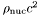], where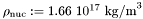Definition at line 387 of file eos.C.

References Lorene::Eos::calcule(), Lorene::Eos::ener_ent_p(), and Lorene::Cmp::get_mp().

## ◆ ener_ent() [2/2]

 Scalar Lorene::Eos::ener_ent ( const Scalar & ent, int nzet, int l_min = 0, Param * par = 0x0 ) const
inherited

Computes the total energy density from the log-enthalpy and extra parameters.

Parameters
 ent [input, unit:] log-enthalpy H defined by, where e is the (total) energy density, p the pressure, n the baryon density, andthe baryon mass nzet number of domains where the energy density is to be computed. l_min index of the innermost domain is which the energy density is to be computed [default value: 0]; the energy density is computed only in domains whose indices are in [l_min,l_min+nzet-1] . In the other domains, it is set to zero. par possible extra parameters of the EOS
Returns
energy density [unit:], whereDefinition at line 397 of file eos.C.

## ◆ ener_ent_p()

 double Lorene::Eos_tabul::ener_ent_p ( double ent, const Param * par = 0x0 ) const
virtual

Computes the total energy density from the log-enthalpy.

Parameters
 ent [input, unit:] log-enthalpy H
Returns
energy density e [unit:], whereImplements Lorene::Eos.

Reimplemented in Lorene::Eos_consistent.

Definition at line 447 of file eos_tabul.C.

References dlpsdlh, Lorene::Tbl::get_taille(), hmax, hmin, Lorene::log10(), logh, logp, and Lorene::pow().

## ◆ eos_from_file() [1/2]

 Eos * Lorene::Eos::eos_from_file ( FILE * fich )
staticinherited

Construction of an EOS from a binary file.

The file must have been created by the function sauve(FILE*) .

Definition at line 193 of file eos_from_file.C.

## ◆ eos_from_file() [2/2]

 Eos * Lorene::Eos::eos_from_file ( ifstream & fich )
staticinherited

Construction of an EOS from a formatted file.

The fist line of the file must start by the EOS number, according to the following conventions:

• 1 = relativistic polytropic EOS (class Eos_poly ).
• 2 = Newtonian polytropic EOS (class Eos_poly_newt ).
• 3 = Relativistic incompressible EOS (class Eos_incomp ).
• 4 = Newtonian incompressible EOS (class Eos_incomp_newt ).
• 5 = Strange matter (MIT Bag model)
• 6 = Strange matter (MIT Bag model) with crust
• 10 = SLy4 (Douchin & Haensel 2001)
- 11 = FPS (Friedman-Pandharipande + Skyrme)
• 12 = BPAL12 (Bombaci et al. 1995)
• 13 = AkmalPR (Akmal, Pandharipande & Ravenhall 1998)
• 14 = BBB2 (Baldo, Bombaci & Burgio 1997)
• 15 = BalbN1H1 (Balberg 2000)
• 16 = GlendNH3 (Glendenning 1985, case 3)
• 17 = Tabulated EOS in CompOSE format
• 18 = magnetized (tabulated) equation of state
• 19 = relativistic ideal Fermi gas at zero temperature (class Eos_Fermi)
• 20 = Tabulated EOS in CompOSE format corrected for thermo. consistency
• 100 = Multi-domain EOS (class MEos )
• 110 = Multi-polytropic EOS (class Eos_multi_poly )
• 120 = Fitted SLy4 (Shibata 2004)
• 121 = Fitted FPS (Shibata 2004)
• 122 = Fitted AkmalPR (Taniguchi 2005)

The second line in the file should contain a name given by the user to the EOS. The following lines should contain the EOS parameters (one parameter per line), in the same order than in the class declaration.

Definition at line 345 of file eos_from_file.C.

## ◆ get_hmax()

 double Lorene::Eos_tabul::get_hmax ( ) const
inline

Returns maximal value of log-enthalpy in the table.

Definition at line 302 of file eos_tabul.h.

References hmax.

## ◆ get_hmin()

 double Lorene::Eos_tabul::get_hmin ( ) const
inline

Returns minimal value of log-enthalpy in the table.

Definition at line 299 of file eos_tabul.h.

References hmin.

## ◆ get_name()

 const char * Lorene::Eos::get_name ( ) const
inherited

Returns the EOS name.

Definition at line 179 of file eos.C.

References Lorene::Eos::name.

## ◆ identify()

 virtual int Lorene::Eos::identify ( ) const
pure virtualinherited

Returns a number to identify the sub-classe of Eos the object belongs to.

## ◆ nbar_ent() [1/2]

 Cmp Lorene::Eos::nbar_ent ( const Cmp & ent, int nzet, int l_min = 0, Param * par = 0x0 ) const
inherited

Computes the baryon density field from the log-enthalpy field and extra parameters.

Parameters
 ent [input, unit:] log-enthalpy H defined by, where e is the (total) energy density, p the pressure, n the baryon density, andthe baryon mass nzet number of domains where the baryon density is to be computed. l_min index of the innermost domain is which the baryon density is to be computed [default value: 0]; the baryon density is computed only in domains whose indices are in [l_min,l_min+nzet-1] . In the other domains, it is set to zero. par possible extra parameters of the EOS
Returns
baryon density [unit: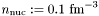]

Definition at line 362 of file eos.C.

References Lorene::Eos::calcule(), Lorene::Cmp::get_mp(), and Lorene::Eos::nbar_ent_p().

## ◆ nbar_ent() [2/2]

 Scalar Lorene::Eos::nbar_ent ( const Scalar & ent, int nzet, int l_min = 0, Param * par = 0x0 ) const
inherited

Computes the baryon density field from the log-enthalpy field and extra parameters.

Parameters
 ent [input, unit:] log-enthalpy H defined by, where e is the (total) energy density, p the pressure, n the baryon density, andthe baryon mass nzet number of domains where the baryon density is to be computed. l_min index of the innermost domain is which the baryon density is to be computed [default value: 0]; the baryon density is computed only in domains whose indices are in [l_min,l_min+nzet-1] . In the other domains, it is set to zero. par possible extra parameters of the EOS
Returns
baryon density [unit:]

Definition at line 372 of file eos.C.

## ◆ nbar_ent_p()

 double Lorene::Eos_tabul::nbar_ent_p ( double ent, const Param * par = 0x0 ) const
virtual

Computes the baryon density from the log-enthalpy.

Parameters
 ent [input, unit:] log-enthalpy H
Returns
baryon density n [unit:]

Implements Lorene::Eos.

Reimplemented in Lorene::Eos_consistent.

Definition at line 419 of file eos_tabul.C.

References dlpsdlh, Lorene::exp(), Lorene::Tbl::get_taille(), hmax, hmin, Lorene::log10(), logh, logp, and Lorene::pow().

## ◆ operator!=()

 virtual bool Lorene::Eos::operator!= ( const Eos & ) const
pure virtualinherited

## ◆ operator==()

 virtual bool Lorene::Eos::operator== ( const Eos & ) const
pure virtualinherited

## ◆ operator>>()

 virtual ostream& Lorene::Eos::operator>> ( ostream & ) const
protectedpure virtualinherited

## ◆ press_ent() [1/2]

 Cmp Lorene::Eos::press_ent ( const Cmp & ent, int nzet, int l_min = 0, Param * par = 0x0 ) const
inherited

Computes the pressure from the log-enthalpy and extra parameters.

Parameters
 ent [input, unit:] log-enthalpy H defined by, where e is the (total) energy density, p the pressure, n the baryon density, andthe baryon mass nzet number of domains where the pressure is to be computed. l_min index of the innermost domain is which the pressure is to be computed [default value: 0]; the pressure is computed only in domains whose indices are in [l_min,l_min+nzet-1] . In the other domains, it is set to zero. par possible extra parameters of the EOS
Returns
pressure [unit:], whereDefinition at line 409 of file eos.C.

## ◆ press_ent() [2/2]

 Scalar Lorene::Eos::press_ent ( const Scalar & ent, int nzet, int l_min = 0, Param * par = 0x0 ) const
inherited

Computes the pressure from the log-enthalpy and extra parameters.

Parameters
 ent [input, unit:] log-enthalpy H defined by, where e is the (total) energy density, p the pressure, n the baryon density, andthe baryon mass nzet number of domains where the pressure is to be computed. l_min index of the innermost domain is which the pressure is to be computed [default value: 0]; the pressure is computed only in domains whose indices are in [l_min,l_min+nzet-1] . In the other domains, it is set to zero. par possible extra parameters of the EOS
Returns
pressure [unit:], whereDefinition at line 419 of file eos.C.

## ◆ press_ent_p()

 double Lorene::Eos_tabul::press_ent_p ( double ent, const Param * par = 0x0 ) const
virtual

Computes the pressure from the log-enthalpy.

Parameters
 ent [input, unit:] log-enthalpy H
Returns
pressure p [unit:], whereImplements Lorene::Eos.

Reimplemented in Lorene::Eos_consistent.

Definition at line 475 of file eos_tabul.C.

References dlpsdlh, Lorene::Tbl::get_taille(), hmax, hmin, Lorene::log10(), logh, logp, and Lorene::pow().

protectedvirtual

Reads the file containing the table and initializes in the arrays logh , logp and dlpsdlh .

Reimplemented in Lorene::Eos_consistent.

Definition at line 258 of file eos_tabul.C.

## ◆ sauve()

 void Lorene::Eos_tabul::sauve ( FILE * fich ) const
virtual

Save in a file.

Reimplemented from Lorene::Eos.

Reimplemented in Lorene::Eos_CompOSE.

Definition at line 245 of file eos_tabul.C.

References Lorene::Eos::sauve(), and tablename.

## ◆ set_name()

 void Lorene::Eos::set_name ( const char * name_i )
inherited

Sets the EOS name.

Definition at line 173 of file eos.C.

References Lorene::Eos::name.

## ◆ Eos::eos_from_file

 Eos* Eos::eos_from_file ( FILE * )
friend

The construction functions from a file.

## ◆ authors

 string Lorene::Eos_tabul::authors
protected

Authors - reference for the table.

Definition at line 194 of file eos_tabul.h.

## ◆ dlpsdlh

 Tbl* Lorene::Eos_tabul::dlpsdlh
protected

Table of.

Definition at line 209 of file eos_tabul.h.

## ◆ dlpsdlnb

 Tbl* Lorene::Eos_tabul::dlpsdlnb
protected

Table of.

Definition at line 215 of file eos_tabul.h.

## ◆ hmax

 double Lorene::Eos_tabul::hmax
protected

Upper boundary of the enthalpy interval.

Definition at line 200 of file eos_tabul.h.

## ◆ hmin

 double Lorene::Eos_tabul::hmin
protected

Lower boundary of the enthalpy interval.

Definition at line 197 of file eos_tabul.h.

## ◆ log_cs2

 Tbl* Lorene::Eos_tabul::log_cs2
protected

Table of.

Definition at line 218 of file eos_tabul.h.

## ◆ logh

 Tbl* Lorene::Eos_tabul::logh
protected

Table of.

Definition at line 203 of file eos_tabul.h.

## ◆ lognb

 Tbl* Lorene::Eos_tabul::lognb
protected

Table of.

Definition at line 212 of file eos_tabul.h.

## ◆ logp

 Tbl* Lorene::Eos_tabul::logp
protected

Table of.

Definition at line 206 of file eos_tabul.h.

## ◆ name

 char Lorene::Eos::name
protectedinherited

EOS name.

Definition at line 212 of file eos.h.

## ◆ tablename

 string Lorene::Eos_tabul::tablename
protected

Name of the file containing the tabulated data.

Definition at line 192 of file eos_tabul.h.

The documentation for this class was generated from the following files: